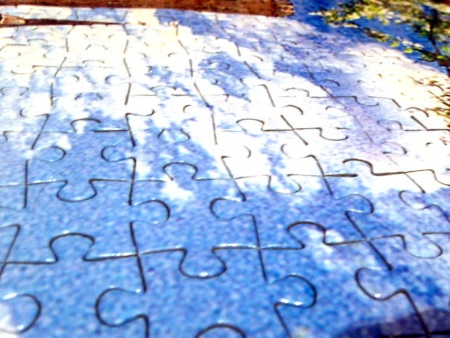# A puzzle puzzle

Jigsaw puzzles that say they have 1,000 pieces have approximately 1,000 pieces, but probably not exactly 1,000. Jigsaw puzzle pieces are typically arranged in a grid, so the number of pieces along a side has to be a divisor of the total number of pieces. This means there aren’t very many ways to make a puzzle with exactly 1,000 pieces, and most have awkward aspect ratios.Since jigsaw pieces are irregularly shaped, it may be surprising to learn that the pieces are actually arranged in a regular grid. At least they usually are. There are exceptions such as circular puzzles or puzzles that throw in a couple small pieces that throw off the grid regularity.

How many aspect ratios can you have with a rectangular grid of 1,000 points? Which ratio comes closest to the golden ratio? More generally, answer the same questions with 10^n points for positive integer n.

More puzzles:

## 7 thoughts on “A puzzle puzzle”

1. Robbie

A grid of 10^n points has (n + 1)^2 possible aspect ratios (Assuming A:B and B:A are each unique), and the closest ratio will be 1:1 (A square of sides 10^(n-1).

2. public class _Main_ {

private java.util.Vector _factors;
private java.util.Iterator _factorsI;
private double _diff;
private int _num;
private int _total;
private int _theOne;
public static final double _GoldenRatio = 1.618034;
public _Main_(int total){
_total = total;
_diff=100;
_factors = new java.util.Vector();
for(int k =1; k<java.lang.Math.sqrt((double)_total);k++){
if(_total%k == 0){
}
}
_num=0;
_factorsI = _factors.iterator();
while(_factorsI.hasNext()){
int temp = _factorsI.next();
if(java.lang.Math.abs(_GoldenRatio-(double)(temp/(_total/temp)))<_diff ||java.lang.Math.abs(_GoldenRatio-(double)((_total/temp)/temp))<_diff){
if(java.lang.Math.abs((double)(temp/(_total/temp)))<java.lang.Math.abs((double)((_total/temp)/temp))){
_diff = java.lang.Math.abs(_GoldenRatio-(double)(temp/(_total/temp)));
}else{
_diff = java.lang.Math.abs(_GoldenRatio-(double)((_total/temp)/temp));
}
_theOne=temp;
}
_num+=1;
}
System.out.println("there are "+_num+" arrangements for "+_total+" pieces.");
System.out.println(_theOne+"x"+_total/_theOne+" is the closest to the golden ratio");
}

public static void main(String[] argvs){
new _Main_(1000);
//There are 8 arrangements for 1000 pieces.
//25×40 is the closest to the golden ratio
}
}

3. Peter

For the generalization of the first question, it’s ceil((n+1)^2/2). The prime factorization of 10 is 2*5, so the prime factorization of 10^n is 2^n * 5^n. For a 2 dimensional jigsaw puzzle, what we want to do is find the number of ways to partition those 2’s and 5’s among the two different axes, so there are n+1 ways to divide up the 2’s, and n+1 ways to divide up the 5’s. However, a 25×40 jigsaw puzzle has the same aspect ratio as a 40×25 puzzle, so you divide by 2, except a 10×10 puzzle is the same as a 10×10 puzzle, so we take the ceiling, since for even n, we would otherwise end up with a fractional number of aspect ratios.

Incidentally, I’m pretty sure most 1000 piece puzzles I’ve done are 25×40, but perhaps I’m misremembering the 1500 piece 30×50 puzzles, which is much closer to phi.

4. For 10^3, 25 x 40 isn’t so bad. I will check the next 1000 piece puzzle I do and report back :)

5. Dave Tate

There are exceptions such as circular puzzles or puzzles that throw in a couple small pieces that throw off the grid regularity.
Actually, quite a few commercial jigsaw puzzles have non-rectangular arrangements, not-fully-interlocking (or even non-interlocking) pieces, and other nefarious ways of making it more difficult to spot a piece’s correct orientation. Of the 2 dozen or so rectangular jigsaw puzzles in my closet, probably about 1/3 depart from a strictly rectangular grid.

6. I prefer Robbie’s point of view that A:B and B:A are unique. I’ll label the following as conjecture for the ratio closest to the golden ratio for some given n.
``` For odd n >= 3:```

``` 40 x 10^(n-3) :: 25 x 10^(n-3) For even n >= 4 ```

```125 x 10^(n-4) :: 80 x 10^(n-4) ```
A proof of this might arise from examining a fraction composed of a bunch of 2’s and 5’s. It doesn’t appear possible to make such a fraction whose value is close to 1.011 or 1.036. That is what would be required to produce a different aspect ratio that is closer to phi than the 40×25 or 125×80 (respectively)